# Descriptive Statistical Measures For Mining In Large Databases

A Descriptive statistic is a statistical summary that quantitatively describes or summarizes features of a collection of information on, while descriptive statistics is the process of using and analyzing those statistics. Descriptive statistics are distinguished from inferential statistics (or inductive statistics) by its aim to summarize a sample.

There are several descriptive statistical measures to mine in large databases in data mining i.e used for knowledge discovery in large databases.

These measures are listed down below.

• Measuring Central Tendency.
• Measuring the Dispersion of Data.
• Boxplot Analysis.
• Visualization of Boxplot Dispersion.
• Histogram Analysis.
• Quantile Plot.
• Quantile-Quantile Plot.
• Scatter Plot.
• Loess Curve.

## Measuring The Central Tendency

### Mean:

• It is the Arithmetic average of the given data.
• For Weighted mean, we use this formula, x=(sum(wi*xi)/sum(wi).

### Median:

• It is a holistic measure of data.
• Given in order, It is nothing but the middlemost value of the dispersed data.
• If there are odd no values then the middle value will be the median.
• If there are even no values then the median is the average of two middle values.
• It can also be estimated by using,

### Mode:

• It is nothing but the value that occurs most frequently in the data.
• If there is only one mode in the data then it is a unimodal data.
• If there are two modes in the data then it is bimodal data.
• If there are three modes in the data then it is trimodal data.
• The empirical formula of mode is, median-mode=3*(mean-median).

## Measuring The Dispersion Of Data

### Quartiles, Outliers, and Boxplots:

• Quartiles: Those are nothing but the 1/4th of the data, Q1 (25th percentile), Q3 (75th percentile).
• Inter-quartile range: It is the differences between the 75th and 25th quartile (IQR = Q3 – Q1).
• Five number summary: It describes five values -> min, Q1, M, Q3, max.
• Boxplot: Ends of the box are the quartiles, the median is marked, whiskers(two lines outside the box extend to Minimum and Maximum) and plot outlier individually.
• Outlier: It is usually, a value higher/lower than 1.5 x IQR

### Variance and standard deviation:

• Variance s2: (algebraic, scalable computation).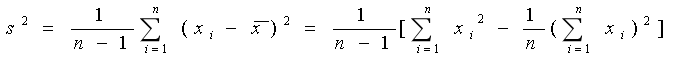• Standard deviation: It is the square root of variance s2

## Boxplot Analysis

• In this type of analysis, visualization of data is represented with a box.
• The ends of the box are at the first and third quartiles, i.e., the height of the box is IRQ.
• The median is marked by a line within the box
• Whiskers: two lines outside the box extend to Minimum and Maximum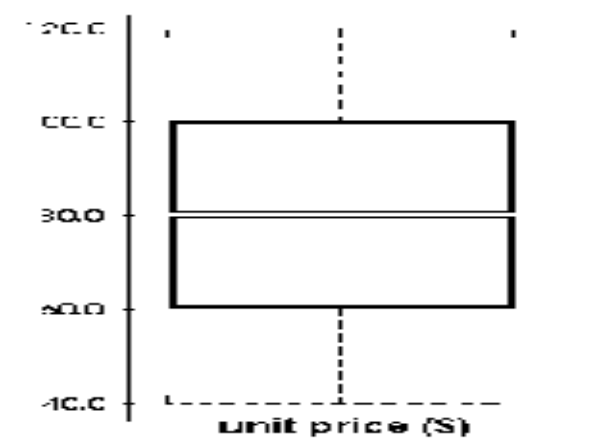## Histogram Analysis

• It is a graph that displays basic statistical class descriptions.
Frequency histograms
• It is a univariate graphical method.
• It consists of a set of rectangles that reflect the counts or frequencies of the classes present in the given data.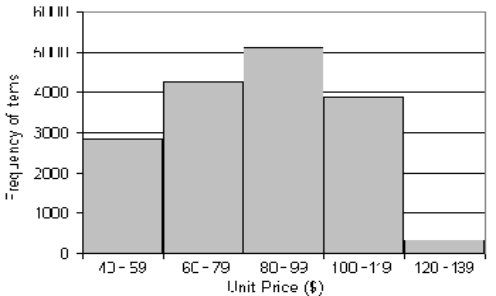## Quantile Plot

• It displays all of the data (allowing the user to assess both the overall behavior and unusual occurrences).
• It plots quantile information
• For a data xi data sorted in increasing order, fi indicates that approximately 100 fi% of the data are below or equal to the value xi.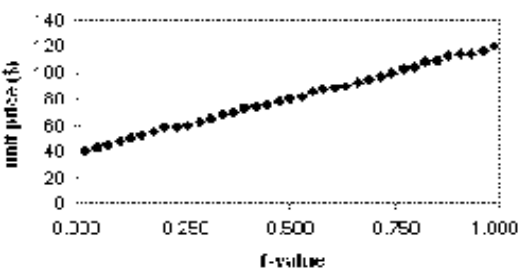## Quantile-Quantile Plot

• It is graphs of the quantiles of one univariate which shows distribution against the corresponding quantiles of another.
• It allows the user to view whether there is a shift in going from one distribution to another.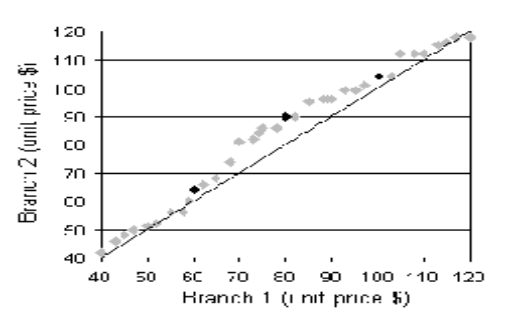## Scatter Plot

• It provides a first look at bivariate data to see clusters of points, outliers, etc.
• Each pair of values is treated as a pair of coordinates and plotted as points in the plane.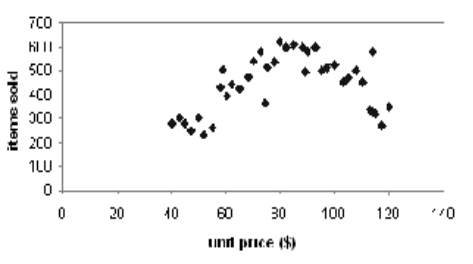## Summary

Descriptive statistical Measure is a statistical summary that quantitatively describes or summarizes features of a collection of information on, while descriptive statistics is the process of using and analyzing those statistics. Descriptive statistics are distinguished from inferential statistics (or inductive statistics) by its aim to summarize a sample.

Subscribe us for more content on Data.